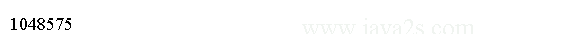# Java Tutorial - Java whole number

## Java byte type

The smallest integer type is `byte`. `byte` type variables are useful when working with a stream of data from a network or file.

Byte variables are declared by use of the byte keyword. The following declares two byte variables called b and c:

`byte b, c;`

`byte` is a signed 8-bit type that has a range from -128 to 127.

The following code creates two byte type variables and assigns values.

```public class Main {
public static void main(String[] args) {
byte b1 = 100;
byte b2 = 20;
System.out.println("Value of byte variable b1 is :" + b1);
System.out.println("Value of byte variable b1 is :" + b2);
}
}
```

The code above generates the following result.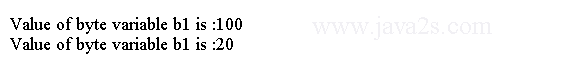The Byte class wraps a value of primitive type byte in an object. Byte class provides several methods for converting a byte to a String and a String to a byte.

## Java short type

The size of Java short type is between byte and integer.

short is a signed 16-bit type. short type variable has a range from -32,768 to 32,767.

Here are some examples of short variable declarations:

```short s;
short t;
```

## Java int type

When byte and short values are used in an expression they are promoted to int when the expression is evaluated.

`int` is a signed 32-bit type that has a range from `-2,147,483,648` to `2,147,483,647`.

## Java long type

Java long type is used when an int type is not large enough.

long is a signed 64-bit type and . The range of long type is -9,223,372,036,854,775,808 to 9,223,372,036,854,775,807

To specify a long literal, you need to tell the compiler that the literal value is of type `long` by appending an upper- or lowercase `L` to the literal. For example, `0x7ffffffffffffffL` or `123123123123L`.

The following code creates a `long` type literal and assigns the value to a `long` type variable.

```public class Main {
public static void main(String args[]) {
long l = 0x7ffffffffffffffL;

System.out.println("l is " + l);
}
}
```

The output generated by this program is shown here: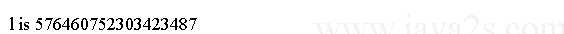## Example

Here is a program that use long type to store the result.

```public class Main {
public static void main(String args[]) {
long result= (long)Integer.MAX_VALUE * (long)10;
System.out.println(result);//21474836470

}
}
```

The result could not have been held in an int variable.

The code above generates the following result.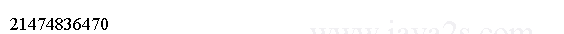## octal integer(base eight)

Octal values are denoted in Java by a leading zero. valid value 09 will produce an error from the compiler, since 9 is outside of octal's 0 to 7 range.

```public class Main {

public static void main(String[] args) {
int i = 010;

System.out.println(i);
}
}
```

The output: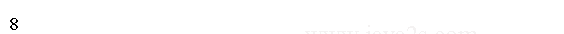hexadecimal matches with modulo 8 word sizes, such as 8, 16, 32, and 64 bits. You signify a hexadecimal constant with a leading zero-x, (0x or 0X).

The range of a hexadecimal digit is 0 to 15, so A through F (or a through f ) are substituted for 10 through 15.

An integer literal can always be assigned to a long variable. An integer can also be assigned to a char as long as it is within range.

```public class Main{
public static void main(String[] argv){
int f = 0XFFFFF;

System.out.println(f);//1048575

}
}
```

The code above generates the following result.